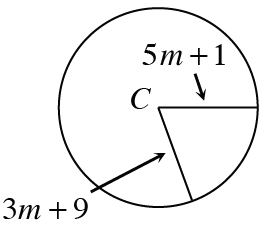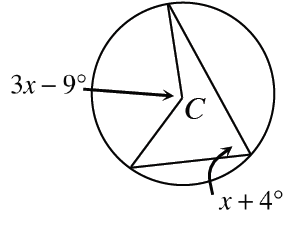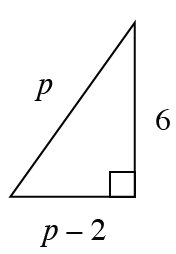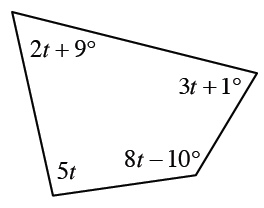### Home > GC > Chapter 10 > Lesson 10.1.2 > Problem10-19

10-19.

For each of the geometric relationships represented below, write and solve an equation for the given variable. For parts (a) and (b), assume that $C$ is the center of the circle. Show all work.

1.Since both are radii, $5m + 1 = 3m + 9$.

$m = 4$

1.The measure of the central angle is twice the measure of the inscribed angle. So $2(x + 4) = 3x - 9$.

1.How are the sides of a right triangle related?

$p = 10$

1.What is the total sum of the angles of this polygon?